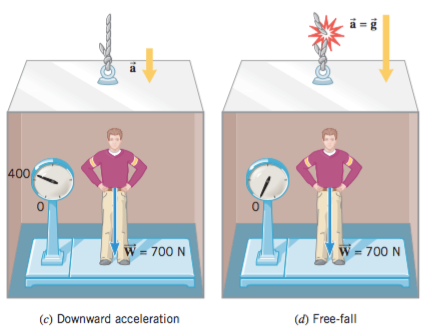top of page
Search
• Yuzhe

# Apparent weight - physics/物理A person whose true weight is 700 N steps on the scale. If the elevator is at rest or moving with a constant velocity (either upward or downward), the scale registers the true weight, as a illustrates. If the elevator is accelerating, the apparent weight and the true weight are not equal. When the elevator accelerates upward, the apparent weight is greater than the true weight, as b shows.Conversely, if the elevator accelerates downward, as in part c, the apparent weight is less than the true weight. In fact, if the elevator falls freely, so its acceleration is equal to the acceleration due to gravity, the apparent weight becomes zero, as part d indicates. In a situation such as this, where the apparent weight is zero, the person is said to be “weightless.” The apparent weight, then, does not equal the true weight if the scale and the

person on it are accelerating.

#Physics

bottom of page